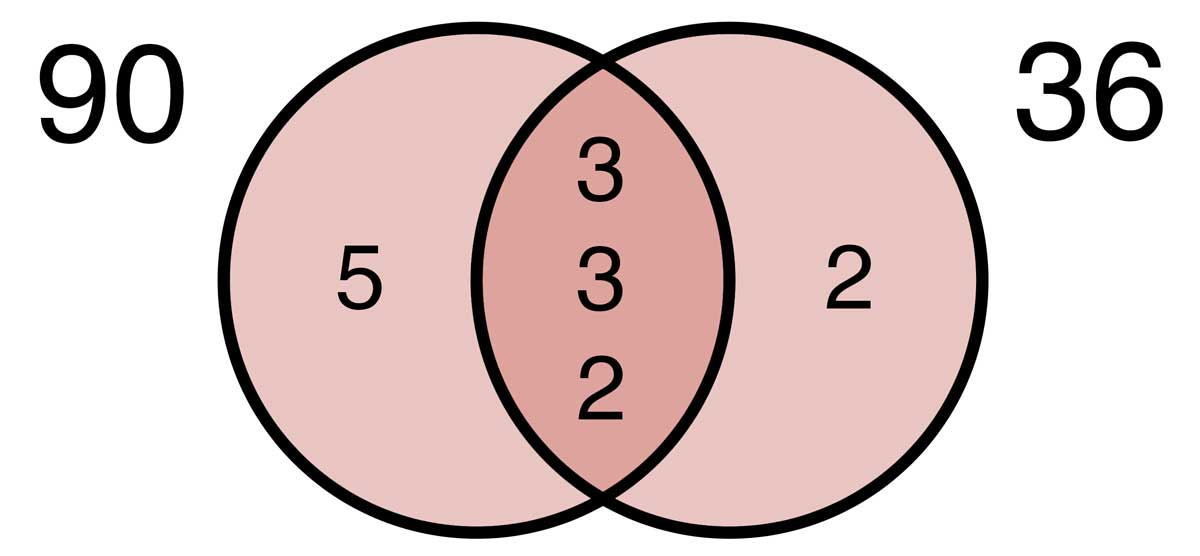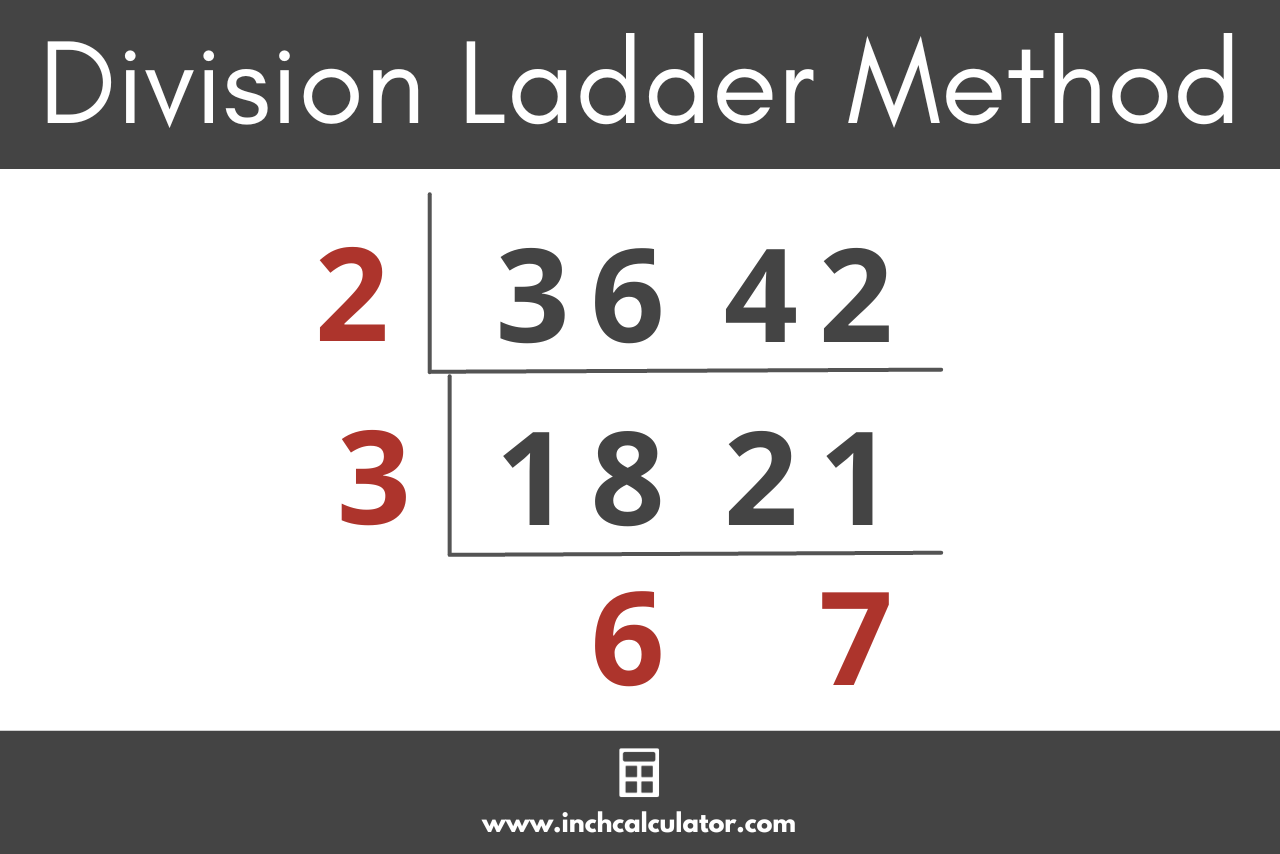# LCM Calculator (Least Common Multiple)

Find the least common multiple for a set of numbers using our LCM calculator below.

Two or more whole numbers, separated by a comma

## Least Common Multiple:

150

### Steps to Solve

150 is the smallest number that is evenly divisible by 15, 25, & 30 without a remainder
150 ÷ 15 = 10
150 ÷ 25 = 6
150 ÷ 30 = 5
Learn how we calculated this below

## How to Find the Least Common Multiple

The least common multiple (LCM), which is sometimes called the lowest common multiple, of a set of numbers is the smallest positive whole number (integer) that can be evenly divided into each one. In other words, the LCM is the smallest number that is a multiple of each value in a set of two or more numbers.

Aside from the calculator above, there are a few methods to find the least common multiple.

### Method One: Find the LCM Using Prime Factorization

One way to find the least common multiple is prime factorization. First, find the prime factors of each number in the set.

Then, multiply all the non-duplicated prime factors to find the least common multiple. That is, find which prime factors are common to all numbers in the set and multiply those factors together, then also multiply any prime factors that are unique to each number in the set.

You can use our prime factorization calculator to find the prime factors for each number.

#### Example: Find the Least Common Multiple of 90 and 36Step one: find the prime factors of 36.

Since 36 is an even number, divide 36 by 2, which equals 18. Since 18 is still even, divide by 2 again to get 9. 9 can be divided by 3 to get 3. Since 3 is prime, we are done. Collecting the divisors we used, we see that the prime factors are thus [3, 3, 2, 2].

Step two: find the prime factors of 90.

Since 90 is also an even number, divide 90 by 2, which equals 45. We know 5 is a prime number that equally divides into 45, so divide 45 by 5 to get 9. As above, 9 can be divided by 3 to get 3. Collecting the divisors we used, we see that the prime factors are thus [5, 3, 3, 2].

Step three: find the prime factors that are common to both 36 and 90 and not common to both 36 and 90.

All of the prime factors of 36 and 90 are [5, 3, 3, 2, 2]. The prime factors common to both 36 and 90 are [3, 3, 2] and the prime factors not common to both 36 and 90 are [5, 2].

This is seen in the Venn diagram above. The prime factor unique to 90 on the left is 5, the prime factor unique to 36 on the right is 2, and the prime factors common to both numbers in the middle are 3, 3, and 2.

Step four: multiply the common and not common factors together to find the least common multiple: 3 x 3 x 2 x 5 x 2.

Note that by being explicit about which factors are common and which are not, we are making sure not to multiply common factors more than once. For example, since 3, 3, and 2 are common between 36 and 90 they are only used once in the multiplication, not twice.

LCM = 5 × 3 × 3 × 3 × 2 × 2
LCM = 180

### Method Two: Find the LCM by Listing the Multiples

You can also find the least common multiple by listing the multiples of each number until you find the smallest multiple that is common to both.

To find multiples of a number, multiply it by 2 to find the first multiple. Then multiply it by 3 to find the second. Continue by multiplying it by 4, and so on to find all of the multiples.

For example, let’s find the least common multiple of 3 and 5 by listing their respective multiples.

The multiples of 3 are [3,6,9,12,15,18,21,24,27,30,…]

The multiples of 5 are [5,10,15,20,25,30,…]

Observe that the multiples in these lists that are common to both 3 and 5 are 15 and 30. The smallest of these is 15, which makes it the least common multiple.

Thus, the least common multiple of 3 and 5 is 15.

### Method Three: Find the LCM using the Division Method

Another method to find the least common multiple is by using the Division Method. In this method, the set of numbers are all divided by a common divisor. The results are then divided by another common divisor.

This process continues until the results no longer have a common divisor among them. Finally, the last results and the divisors are all multiplied together to find the least common multiple.

For example, let’s find the LCM of 30 and 36. They are both even numbers, so we know 2 is a common divisor:

30 ÷ 2 = 15
36 ÷ 2 = 18

Now, we know that 3 is a common divisor of 15 and 18:

15 ÷ 3 = 5
18 ÷ 3 = 6

Since 5 and 6 do not have any common divisors (other than 1), we are done dividing. Finally we take our final results, 5 and 6, and multiply them together, along with our divisors, 2 and 3, to get our least common multiple.

LCM = 2 × 3 × 5 × 6 = 180

So, the least common multiple of 30 and 36 is 180.

### Method Four: Find the LCM using the Cake/Ladder Method

The Cake, or Ladder, Method for finding the least common multiple is actually the same as the Division Method, just with a different visual representation. In this method, the two (or more) numbers for which we want to find the LCM are placed in a division box, and a common divisor is used to divide them.

Another box is then drawn around the results which are then divided by a common divisor again. This continues until the remaining values have no common divisors. The LCM is then found by simply multiplying all the values outside the boxes.

For example, let’s find the LCM of 36 and 42 using the Cake/Ladder Method.LCM = 2 × 3 × 6 × 7 = 252

As we can see, 36 and 42 have a common divisor of 2 which divides them into 18 and 21, respectively. 18 and 21 have a common divisor of 3 which divides them into 6 and 7, respectively. 6 and 7 do not have a common divisor other than 1, so no more dividing takes place.

We now see that 2, 3, 6, and 7 are all outside the box, so we multiply them together to find 252, the value of the least common multiple of 36 and 42.

### Method Five: Find the LCM using the Greatest Common Factor (GCF) Method

This is a very simple method for finding the LCM of a set of numbers. If you already know the greatest common factor of the set of numbers, then you can use the GCF Method for finding the least common multiple.

To do so, simply divide each number by the GCF. Then, take the resulting values and multiply them together, along with the GCF and that’s your least common multiple of the set!

For example, let’s find the LCM of 21 and 49 using the GCF Method. We already know that the GCF of 21 and 49 is 7, so let’s divide both by 7 and multiply 7 by the results:

21 ÷ 7 = 3
49 ÷ 7 = 7

LCM = 7 × 3 × 7 = 147

Therefore, the least common multiple of 21 and 49 is 147.